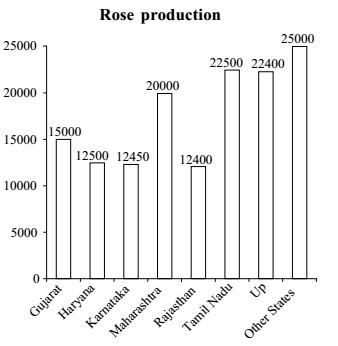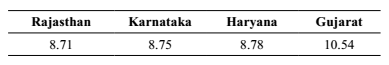## Introduction to Data Interpretation

#### Data Interpretation

Direction: Study the following graph carefully and answer the questions given below it.1. What is the approximate average production of roses (in thousands) across all the states?

1. Total production of rose by all the States = 142250
Total number of states = 8

##### Correct Option: D

Total production of rose by all the States = 142250
Total number of states = 8
Average = Total production of rose by all the States / Total number of states

 ∴ Average = 142250 ≈ 18 thousand 8 x 1000

1. Which of the following State(s) contribute(s) less than 10 % in the total rose production?

1. Total rose production = ( 15000 + 12500 + 12450 + 20000 + 12400 + 22500 + 22400 + 25000 ) = 142250
Calculate the % rose production.
% Rose Production increase in State = Rose production in state x 100 / Rose production in All state
Percentage production of rose in the States (the lowest four states)

##### Correct Option: C

Total rose production = ( 15000 + 12500 + 12450 + 20000 + 12400 + 22500 + 22400 + 25000 ) = 142250
% Rose Production increase in State = Rose production in state x 100 / Rose production in All state
% Rose Production increase in Gujrat = Rose production in Gujrat x 100 / Rose production in All state
% Rose Production increase in Gujrat = 15000 x 100 / 142250
% Rose Production increase in Gujrat = 1500 x 100 / 14225
% Rose Production increase in Gujrat = 300 x 100 / 285
% Rose Production increase in Gujrat = 60 x 100 / 57
% Rose Production increase in Gujrat = 6000 / 57 = 10.54 %
Similarly Calculate the % Rose Production increase in all states one by one and compare them.

Percentage production of rose in the States (the lowest four states)1. If total percentage contribution of the States having production of roses below twenty thousand is considered, which of the following statements is true?

1. It is 36.8% approximately.

##### Correct Option: E

It is 36.8% approximately.

1. By what percentage rose production of other States is more than that of the Maharashtra?

1. Required percentage

 = 25 - 20 X 100 20

##### Correct Option: A

Required percentage

 = 25 - 20 X 100 = 25 % (more) 20

1. Approximately what percentage of the total rose production is shared by the other States?

1. Required percentage

 = 25 X 100 ≈ 20% 142.25

##### Correct Option: B

Required percentage

 = 25 X 100 ≈ 20% 142.25